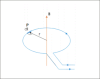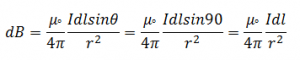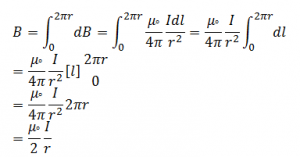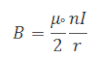## TyroCity

Physics XII Notes for Physics Notes

Posted on • Updated on

# Magnetic Field Along The Centre Of Circular Current Carrying CoilConsider a circular current carrying coil having radius r and centre O. When the current is passing through the circular coil, magnetic field is produced. To find the magnetic field at the centre of the circular coil, consider a length of element dl at point p which is tangent to the circular coil. The angle between element dl and radius r is 90°.
According to the biot-savart law, the magnetic field at the centre of the circular coil due to element dl is:Total magnetic field due to the circular coil is:If there are n number of circular coil then their magnetic field is: